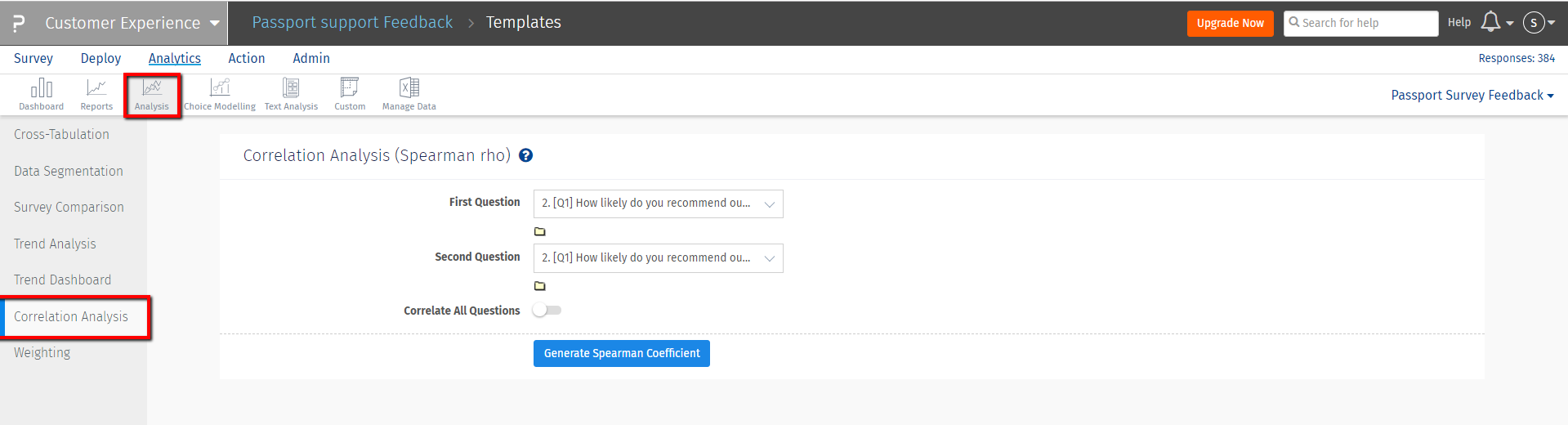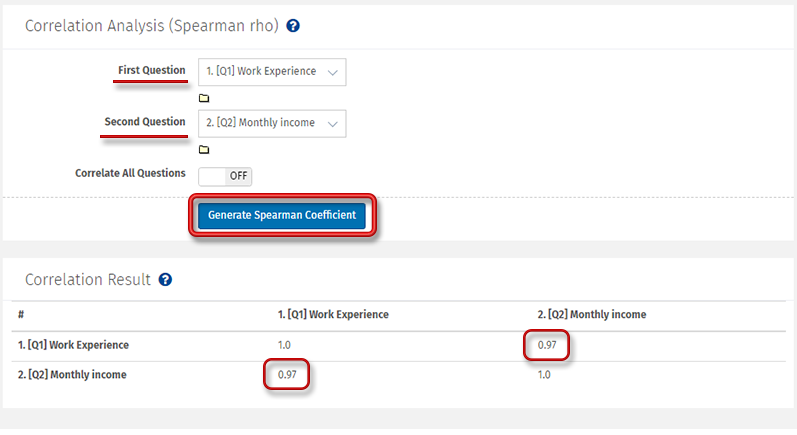# CX - Correlation Analysis (Spearman rho) - FAQ?

Correlation is a bivariate analysis that measures the strength of association between two variables and the direction of the relationship.

In terms of the strength of relationship, the value of the correlation coefficient (rs) varies between+1 and -1. As the correlation coefficient value goes towards 0, the relationship between the two variables will be weaker.

The direction of the relationship is indicated by the sign of the coefficient; a + sign indicates a positive relationship and a – sign indicates a negative relationship.

In general,
• rs > 0 implies positive agreement among ranks
• rs < 0 implies negative agreement (or agreement in the reverse direction)
• rs = 0 implies no agreement
Spearman rank correlation: It is a non-parametric test that is used to measure the degree of association between two variables. The Spearman rank correlation test does not carry any assumptions about the distribution of the data.

The following formula is used to calculate the Spearman rank correlation:• rs = Spearman rank correlation
• D = the difference between the ranks of corresponding variables
• n = number of observations

## How can I run correlation analysis for my survey?

Go to: Login » Customer Experience » Analytics » Analysis » Correlation AnalysisTo Correlate:
• Select the first and the second question that you wish to correlate. You can also select all questions.
• Click on Generate Spearman Coefficient button to generate the report.In the above example we find out the relationship between two variables Work Experience and Monthly Income.

In general, we know that Monthly Income increases with the Work Experience. This means there should be positive association between these two variables which is proved by rs value which is 0.97.

This implies there is very strong association between the two variables. Any increase in one variable leads to increase in other.

## What question types are supported for Correlation Analysis?

All select type questions are supported for correlation analysis.